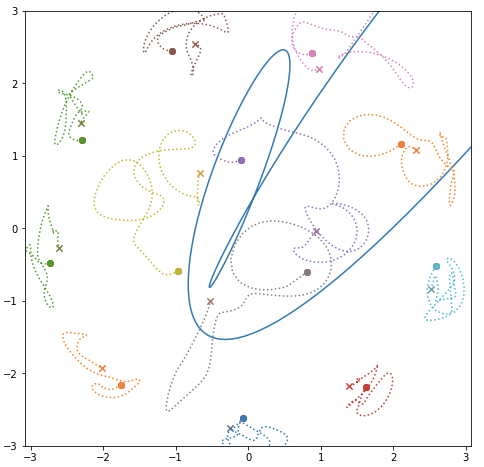# Shuffling the sheep

$$\def\xx{\mathbf{x}}\def\Real{\mathbb{R}}$$

We consider the problem of flock control: what are the natural constraints on the steering agents in reconfiguring an ensemble of agents?

Our basic setup is following: the agents are modeled as points $$\xx=(x_1,\ldots,x_n), x_k\in X, k=1,\ldots, N$$, with $$X$$ some terrain (for simplicity, we constrain ourselves here to Euclidean plane, but in general it can be some reasonably tame subset of a manifold). Quasi-static behavior is assumed, under which the system, for each value of the controlling parameters quickly settles into (locally minimizing) the equilibrium whose basin of attraction contains current position.

We will assume that the mutual interactions between the agents in the flock are governed by the interaction term of the energy
$$R(\xx)=\sum_{k\neq l} R(x_k, x_l).$$

Here $$R$$ is a repulsive term forcing the agents to avoid each other. For the case of planar terrain, Coulomb interactions are especially attractive options, thanks to connections to potential theory and related holomorphic parameterizations:
$$R(x_k,x_l)=-\log |x_k-x_l|.$$

To keep the agent confined, we impose a global term,
$$U_0(\xx)=\sum_k U_0(x_k).$$
Here $$U$$ is a coercive function ($$U(x_k):=\alpha |x_k|^2$$ is an obvious choice).

Last element of the setup, is the controller term, – or, rather, an explicit dependence of the global energy sector on some control parameters.

We consider here two versions:

1. The steering agent is herself embedded into the terrain, and her contribution to the potential also has the repulsive force structure:
$$U_p(x_k)=U_0(x_k)-K\log|p-x_k), p\in X.$$
We will be referring to this setting as the “shepherd” setting.
2. The confining potential function $$U_p$$ is made explicitly dependent on the parameter $$p\in P$$, some space of parameters. As a non-example, we can consider $$U_p(x)=U_0(x)+\langle p, x\rangle$$. More interesting would be to make the quadratic form $$U$$ dependent on the parameter running through positive definite forms.

As we indicated above, the overall dynamics is assumed to be fast on the agents, settling to their equilibrium state corresponding to the fixed values of the steering parameter $$p$$, and slow for the parameter $$p$$. This leads one to the following formulation:

$$\dot{x}_k=-\frac{1}{\epsilon}\frac{\partial U_p(\xx)}{\partial x_k},\\ U_p(\xx)=\sum_k \left( U_p(x_k)+\sum_{l\neq k} R(x_k,x_l)\right),\\ \dot{p}=u, u\in U.$$
Intuitively, the model corresponds to Ginibre ensemble (eigenvalues of Gaussian complex matrices), with a perturbative term (corresponding to “shepherd”).

#### A Simulation Study (credits: E. Yarkony)

Agents positions $$x(t)=(x_k(t))_{k=1}^M$$, “shepherd” (steering agent) trajectory $$p(t)$$.

Potential function:
$$U(x,p) = \frac{\alpha}{2}\|x\|^2 + \frac{1}{2}\sum_{k\neq j} \log\|x_k-x_j\|^2 + \frac{1}{2}\sum_k\log\|x_k-p\|^2.$$

Dog trajectory initial and final condition: $$p(0)=p(T)=p_0$$
Agents initial condition at equilibrium: $$\nabla U(x(0),p(0))=0$$
Temporal evolution:
$$\dot x(t) = -\nabla U(x(t),p(t))$$

The gradient (for each agent agent) is:
\begin{align} \nabla_{x_k} U(x,p) &= \nabla \frac{\alpha}{2}\|x_k\|^2 + \frac{1}{2}\sum_{j\neq k} \nabla_{x_k}\log\|x_k-x_j\|^2 + \frac{1}{2} \nabla_{x_k}\log\|x_k-p\|^2\\ &= \alpha x + \sum_{k\neq j} \frac{x_k-x_j}{\|x_k-x_j\|^2} + \frac{x_k-p}{\|x_k-p\|^2} \end{align}

Example of simulation with herd of 12:One can see starting (dot) and ending (cross) positions of the agents. The trajectory of the steering agent is solid blue. The agents start and end in an equilibrium position; n3o braiding of the agents observed.

#### Some natural questions to start:

1. Assume the first, “shepherd”, model. Fix a (smooth) trajectory $$p:[0,T]\to X\equiv \Real^2$$, starting and ending at the same point $$p_0$$ far away from the origin. This loop would generate a movement among the charges $$x_k, k=1,\ldots,n$$.Do the point return to the same positions reshuffled?
2. When they do, one generates so called braid, an element in the fundamental group of the configuration space of $$n$$ (indistinguishable) points in plane. What elements can be generated?
3. What elements can be generated if the movements of the shepherd are constrained, for example to stay outside of the convex hull of the agents?